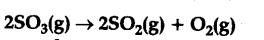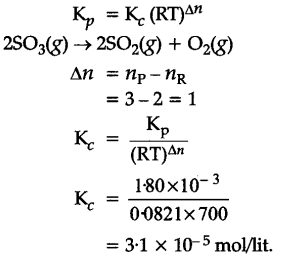# At 700 K, the equilibrium constant \${{K}_{p}}\$ for the reaction

At 700 K, the equilibrium constant {{K}_{p}} for the reactionis (1-80 x {{10}^{-3}} K\${{P}_{a}}\$). What is the numerical value in moles per litre of IQ for this reaction at the same temperature ?